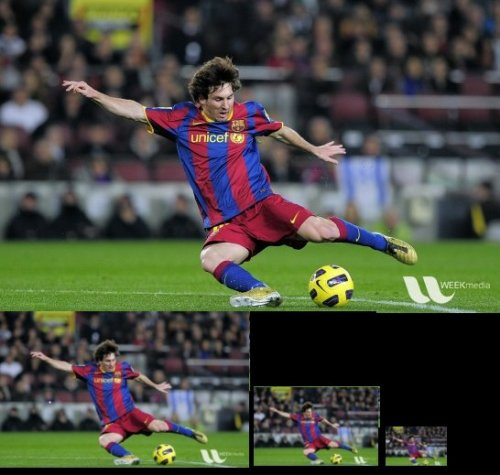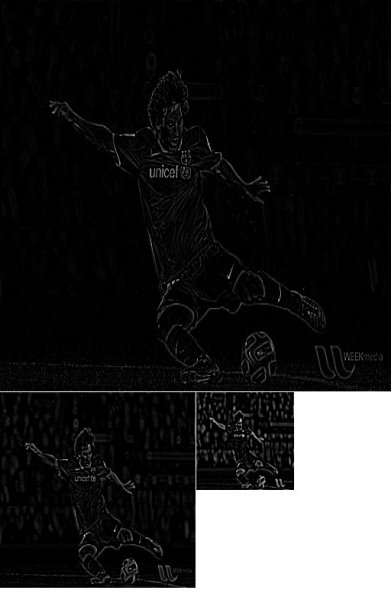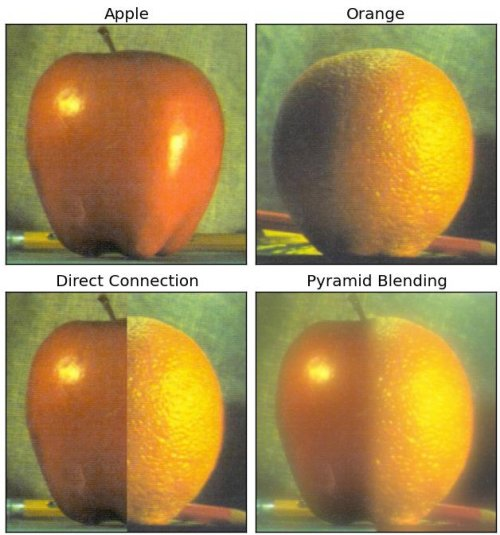# OpenCV-Python官方文档中文翻译20：Image Pyramids

Image Pyramids

## Goal

In this chapter,

• We will learn about Image Pyramids
• We will use Image pyramids to create a new fruit, “Orapple”
• We will see these functions: cv.pyrUp(), cv.pyrDown()
• 关于图像金字塔
• 使用图像金字塔创建一个新的水果"Orapple"
• 函数 cv.pyrUp(),cv.pyrDown()

## Theory

Normally, we used to work with an image of constant size. But on some occasions, we need to work with (the same) images in different resolution. For example, while searching for something in an image, like face, we are not sure at what size the object will be present in said image. In that case, we will need to create a set of the same image with different resolutions and search for object in all of them. These set of images with different resolutions are called Image Pyramids (because when they are kept in a stack with the highest resolution image at the bottom and the lowest resolution image at top, it looks like a pyramid).

There are two kinds of Image Pyramids. 1) Gaussian Pyramid and 2) Laplacian Pyramids

Higher level (Low resolution) in a Gaussian Pyramid is formed by removing consecutive rows and columns in Lower level (higher resolution) image. Then each pixel in higher level is formed by the contribution from 5 pixels in underlying level with gaussian weights. By doing so, a M×N image becomes M/2×N/2 image. So area reduces to one-fourth of original area. It is called an Octave. The same pattern continues as we go upper in pyramid (ie, resolution decreases). Similarly while expanding, area becomes 4 times in each level. We can find Gaussian pyramids using cv.pyrDown() and cv.pyrUp() functions.

img = cv.imread("messi5.jpg")
lower_reso = cv.pyrDown(higher_reso)


Below is the 4 levels in an image pyramid.Now you can go down the image pyramid with cv.pyrUp() function.

higher_reso2 = cv.pyrUp(lower_reso)


Remember, higher_reso2 is not equal to higher_reso, because once you decrease the resolution, you loose the information. Below image is 3 level down the pyramid created from smallest image in previous case. Compare it with original image:

[外链图片转存失败,源站可能有防盗链机制,建议将图片保存下来直接上传(img-EU86BTEw-1614271372859)(https://docs.opencv.org/4.5.1/messiup.jpg)]

Laplacian Pyramids are formed from the Gaussian Pyramids. There is no exclusive function for that. Laplacian pyramid images are like edge images only. Most of its elements are zeros. They are used in image compression. A level in Laplacian Pyramid is formed by the difference between that level in Gaussian Pyramid and expanded version of its upper level in Gaussian Pyramid. The three levels of a Laplacian level will look like below (contrast is adjusted to enhance the contents):## Image Blending using Pyramids使用金字塔来进行图像融合

One application of Pyramids is Image Blending. For example, in image stitching, you will need to stack two images together, but it may not look good due to discontinuities between images. In that case, image blending with Pyramids gives you seamless blending without leaving much data in the images. One classical example of this is the blending of two fruits, Orange and Apple. See the result now itself to understand what I am saying:Please check first reference in additional resources, it has full diagramatic details on image blending, Laplacian Pyramids etc. Simply it is done as follows:

1. Load the two images of apple and orange
2. Find the Gaussian Pyramids for apple and orange (in this particular example, number of levels is 6)
3. From Gaussian Pyramids, find their Laplacian Pyramids
4. Now join the left half of apple and right half of orange in each levels of Laplacian Pyramids
5. Finally from this joint image pyramids, reconstruct the original image.
• 加载苹果和橘子的图片
• 找到苹果和橘子的高斯金字塔
• 从高斯金字塔，找到拉普拉斯金字塔
• 在每个拉普拉斯金字塔的级别中加入苹果的左半边和橘子的右半边
• 最后从这个联合图像的金字塔重建初图像

Below is the full code. (For sake of simplicity, each step is done separately which may take more memory. You can optimize it if you want so).

import cv2 as cv
import numpy as np,sys
A = cv.imread('apple.jpg')
B = cv.imread('orange.jpg')
#给A 生成高斯金字塔
G = A.copy()
gpA = [G]
for i in xrange(6):
G = cv.pyrDown(G)
gpA.append(G)
#为B 生成高斯金字塔
G = B.copy()
gpB = [G]
for i in xrange(6)
G = cv.pyrDown(G)
gpB.append(G)
#给A生成拉普拉斯金字塔
lpA = [gpA]
for i in xrange(5,0,-1):
GE = cv.pyrUp(gpA[i])
L = cv.subtract(gpA[i-1],GE)
lpA.append(L)
# generate Laplacian Pyramid for B
lpB = [gpB]
for i in xrange(5,0,-1):
GE = cv.pyrUp(gpB[i])
L = cv.subtract(gpB[i-1],GE)
lpB.append(L)
# Now add left and right halves of images in each level

LS = []

for la,lb in zip(lpA,lpB):

rows,cols,dpt = la.shape
ls = np.hstack((la[:,0:cols/2], lb[:,cols/2:]))

LS.append(ls)
# now reconstruct

ls_ = LS

for i in xrange(1,6):
ls_ = cv.pyrUp(ls_)
ls_ = cv.add(ls_,LS[i])
#image with direct connecting each half
real = np.hstack((A[:,:cols/2],B[:,cols/2:]))
cv.imwrite("Pyramid_blending2.jpg",ls_)
cv.imwrite('Direct_blending.jpg',real)



## Additional Resources

1. Image Blending

## Exercises

03-2611110-13337
11-1299
08-01269
10-24174
05-0274
03-07186
12-2063
11-28127
04-13889
12-04141
03-11302
11-26421
01-151213
03-27254
07-1284
12-2015
12-0728
02-2995
©️2020 CSDN 皮肤主题: 深蓝海洋 设计师:CSDN官方博客